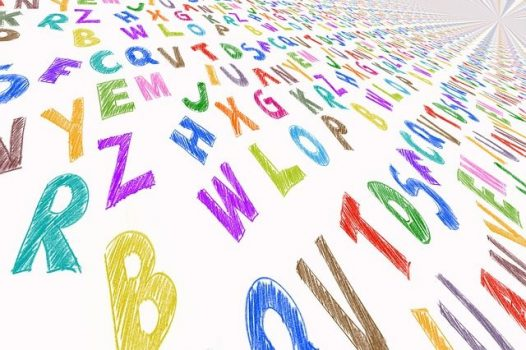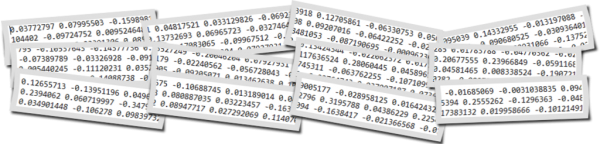## Text Clustering with doc2vec Word Embedding Machine Learning ModelIn this post we will look at doc2vec word embedding model, how to build it or use pretrained embedding file. For practical example we will explore how to do text clustering with doc2vec model.

## Doc2vec

Doc2vec is an unsupervised computer algorithm to generate vectors for sentence/paragraphs/documents. The algorithm is an adaptation of word2vec which can generate vectors for words. Below you can see frameworks for learning word vector word2vec (left side) and paragraph vector doc2vec (right side). For learning doc2vec, the paragraph vector was added to represent the missing information from the current context and to act as a memory of the topic of the paragraph. 

If you need information about word2vec here are some posts:
word2vec –
Vector Representation of Text – Word Embeddings with word2vec
word2vec application –
Text Analytics Techniques with Embeddings
Using Pretrained Word Embeddinigs in Machine Learning
K Means Clustering Example with Word2Vec in Data Mining or Machine Learning

The vectors generated by doc2vec can be used for tasks like finding similarity between sentences / paragraphs / documents.  With doc2vec you can get vector for sentence or paragraph out of model without additional computations as you would do it in word2vec, for example here we used function to go from word level to sentence level:
Text Clustering with Word Embedding in Machine Learning

word2vec was very successful and it created idea to convert many other specific texts to vector. It can called “anything to vector”. So there are many different word embedding models that like doc2vec can convert more than one word to numeric vector.  Here are few examples:

tweet2vec Tweet2Vec: Character-Based Distributed Representations for Social Media
lda2vec Mixing Dirichlet Topic Models and Word Embeddings to Make lda2vec. Here is proposed model that learns dense word vectors jointly with Dirichlet-distributed latent document-level mixtures of topic vectors.
Topic2Vec Learning Distributed Representations of Topics
Med2vec Multi-layer Representation Learning for Medical Concepts
The list can go on. In the next section we will look how to load doc2vec and use for text clustering.

## Building doc2vec Model

Here is the example for converting word paragraph to vector using own built doc2vec model. The example is taken from .

The script consists of the following main steps:

• build model using own text
• save model to file
• load model from this file
• infer vector representation
```
from gensim.test.utils import common_texts
from gensim.models.doc2vec import Doc2Vec, TaggedDocument

print (common_texts)

"""
output:
[['human', 'interface', 'computer'], ['survey', 'user', 'computer', 'system', 'response', 'time'], ['eps', 'user', 'interface', 'system'], ['system', 'human', 'system', 'eps'], ['user', 'response', 'time'], ['trees'], ['graph', 'trees'], ['graph', 'minors', 'trees'], ['graph', 'minors', 'survey']]
"""

documents = [TaggedDocument(doc, [i]) for i, doc in enumerate(common_texts)]

print (documents)
"""
output
[TaggedDocument(words=['human', 'interface', 'computer'], tags=), TaggedDocument(words=['survey', 'user', 'computer', 'system', 'response', 'time'], tags=), TaggedDocument(words=['eps', 'user', 'interface', 'system'], tags=), TaggedDocument(words=['system', 'human', 'system', 'eps'], tags=), TaggedDocument(words=['user', 'response', 'time'], tags=), TaggedDocument(words=['trees'], tags=), TaggedDocument(words=['graph', 'trees'], tags=), TaggedDocument(words=['graph', 'minors', 'trees'], tags=), TaggedDocument(words=['graph', 'minors', 'survey'], tags=)]

"""

model = Doc2Vec(documents, size=5, window=2, min_count=1, workers=4)
#Persist a model to disk:

from gensim.test.utils import get_tmpfile
fname = get_tmpfile("my_doc2vec_model")

print (fname)
#output: C:\Users\userABC\AppData\Local\Temp\my_doc2vec_model

model.save(fname)
# you can continue training with the loaded model!
#If you’re finished training a model (=no more updates, only querying, reduce memory usage), you can do:

model.delete_temporary_training_data(keep_doctags_vectors=True, keep_inference=True)

#Infer vector for a new document:
#Here our text paragraph just 2 words
vector = model.infer_vector(["system", "response"])
print (vector)

"""
output

[-0.08390492  0.01629403 -0.08274432  0.06739668 -0.07021132]

"""
```

## Using Pretrained doc2vec Model

We can skip building embedding file step and use already built file. Here is an example how to do coding with pretrained word embedding file for representing test docs as vectors. The script is based on .

The below script is using pretrained on Wikipedia data doc2vec model from this location

Here is the link where you can find links to different pre-trained doc2vec and word2vec models and additional information.

You need to download zip file, unzip , put 3 files at some folder and provide path in the script. In this example it is “doc2vec/doc2vec.bin”

The main steps of the below script consist of just load doc2vec model and infer vectors.

```
import gensim.models as g
import codecs

model="doc2vec/doc2vec.bin"
test_docs="data/test_docs.txt"
output_file="data/test_vectors.txt"

#inference hyper-parameters
start_alpha=0.01
infer_epoch=1000

test_docs = [ x.strip().split() for x in codecs.open(test_docs, "r", "utf-8").readlines() ]

#infer test vectors
output = open(output_file, "w")
for d in test_docs:
output.write( " ".join([str(x) for x in m.infer_vector(d, alpha=start_alpha, steps=infer_epoch)]) + "\n" )
output.flush()
output.close()

"""
output file
0.03772797 0.07995503 -0.1598981 0.04817521 0.033129826 -0.06923918 0.12705861 -0.06330753 .........
"""
```

So we got output file with vectors (one per each paragraph). That means we successfully converted our text to vectors. Now we can use it for different machine learning algorithms such as text classification, text clustering and many other. Next section will show example for Birch clustering algorithm with word embeddings.

## Using Pretrained doc2vec Model for Text Clustering (Birch Algorithm)

In this example we use Birch clustering algorithm for clustering text data file from 
Birch is unsupervised algorithm that is used for hierarchical clustering. An advantage of this algorithm is its ability to incrementally and dynamically cluster incoming data 

We use the following steps here:

• Load text docs that will be clustered
• Convert docs to vectors (infer_vector)
• Do clustering
```from sklearn import metrics

import gensim.models as g
import codecs

model="doc2vec/doc2vec.bin"
test_docs="data/test_docs.txt"

#inference hyper-parameters
start_alpha=0.01
infer_epoch=1000

test_docs = [ x.strip().split() for x in codecs.open(test_docs, "r", "utf-8").readlines() ]

print (test_docs)
"""
[['the', 'cardigan', 'welsh', 'corgi'........
"""

X=[]
for d in test_docs:

X.append( m.infer_vector(d, alpha=start_alpha, steps=infer_epoch) )

k=3

from sklearn.cluster import Birch

brc = Birch(branching_factor=50, n_clusters=k, threshold=0.1, compute_labels=True)
brc.fit(X)

clusters = brc.predict(X)

labels = brc.labels_

print ("Clusters: ")
print (clusters)

silhouette_score = metrics.silhouette_score(X, labels, metric='euclidean')

print ("Silhouette_score: ")
print (silhouette_score)

"""
Clusters:
[1 0 0 1 1 2 1 0 1 1]
Silhouette_score:
0.17644188
"""

```

If you want to get some test with text clustering and word embeddings here is the online demo Currently it is using word2vec and glove models and k means clustering algorithm. Select ‘Text Clustering’ option and scroll down to input data.

## Conclusion

We looked what is doc2vec is, we investigated 2 ways to load this model: we can create embedding model file from our text or use pretrained embedding file. We applied doc2vec to do Birch algorithm for text clustering. In case we need to work with paragraph / sentences / docs, doc2vec can simplify word embedding for converting text to vectors.

## Text Clustering with Word Embedding in Machine LearningText clustering is widely used in many applications such as recommender systems, sentiment analysis, topic selection, user segmentation. Word embeddings (for example word2vec) allow to exploit ordering
of the words and semantics information from the text corpus. In this blog you can find several posts dedicated different word embedding models:

In contrast to last post from the above list, in this post we will discover how to do text clustering with word embeddings at sentence (phrase) level. The sentence could be a few words, phrase or paragraph like tweet. For examples we have 1000 of tweets and want to group in several clusters. So each cluster would contain one or more tweets.

## Data

Our data will be the set of sentences (phrases) containing 2 topics as below:
Note: I highlighted in bold 3 sentences on weather topic, all other sentences have totally different topic.
sentences = [[‘this’, ‘is’, ‘the’, ‘one’,’good’, ‘machine’, ‘learning’, ‘book’],
[‘this’, ‘is’, ‘another’, ‘book’],
[‘one’, ‘more’, ‘book’],
[‘weather’, ‘rain’, ‘snow’],
[‘yesterday’, ‘weather’, ‘snow’],
[‘forecast’, ‘tomorrow’, ‘rain’, ‘snow’],

[‘this’, ‘is’, ‘the’, ‘new’, ‘post’],
[‘this’, ‘is’, ‘about’, ‘more’, ‘machine’, ‘learning’, ‘post’],
[‘and’, ‘this’, ‘is’, ‘the’, ‘one’, ‘last’, ‘post’, ‘book’]]

## Word Embedding Method

For embeddings we will use gensim word2vec model. There is also doc2vec model – but we will use it at next post.
With the need to do text clustering at sentence level there will be one extra step for moving from word level to sentence level. For each sentence from the set of sentences, word embedding of each word is summed and in the end divided by number of words in the sentence. So we are getting average of all word embeddings for each sentence and use them as we would use embeddings at word level – feeding to machine learning clustering algorithm such k-means.

Here is the example of the function that doing this:

```def sent_vectorizer(sent, model):
sent_vec =[]
numw = 0
for w in sent:
try:
if numw == 0:
sent_vec = model[w]
else:
numw+=1
except:
pass

return np.asarray(sent_vec) / numw
```

Now we will use text clustering Kmeans algorithm with word2vec model for embeddings. For kmeans algorithm we will use 2 separate implementations with different libraries NLTK for KMeansClusterer and sklearn for cluster. This was described in previous posts (see the list above).

The code for this article can be found in the end of this post. We use 2 for number of clusters in both k means text clustering algorithms.
Additionally we will plot data using tSNE.

## Output

Below are results

[1, 1, 1, 0, 0, 0, 1, 1, 1]

Cluster id and sentence:
1:[‘this’, ‘is’, ‘the’, ‘one’, ‘good’, ‘machine’, ‘learning’, ‘book’]
1:[‘this’, ‘is’, ‘another’, ‘book’]
1:[‘one’, ‘more’, ‘book’]
0:[‘weather’, ‘rain’, ‘snow’]
0:[‘yesterday’, ‘weather’, ‘snow’]
0:[‘forecast’, ‘tomorrow’, ‘rain’, ‘snow’]

1:[‘this’, ‘is’, ‘the’, ‘new’, ‘post’]
1:[‘this’, ‘is’, ‘about’, ‘more’, ‘machine’, ‘learning’, ‘post’]
1:[‘and’, ‘this’, ‘is’, ‘the’, ‘one’, ‘last’, ‘post’, ‘book’]

Score (Opposite of the value of X on the K-means objective which is Sum of distances of samples to their closest cluster center):
-0.0008175040203510163
Silhouette_score:
0.3498247

Cluster id and sentence:
1 [‘this’, ‘is’, ‘the’, ‘one’, ‘good’, ‘machine’, ‘learning’, ‘book’]
1 [‘this’, ‘is’, ‘another’, ‘book’]
1 [‘one’, ‘more’, ‘book’]
0 [‘weather’, ‘rain’, ‘snow’]
0 [‘yesterday’, ‘weather’, ‘snow’]
0 [‘forecast’, ‘tomorrow’, ‘rain’, ‘snow’]

1 [‘this’, ‘is’, ‘the’, ‘new’, ‘post’]
1 [‘this’, ‘is’, ‘about’, ‘more’, ‘machine’, ‘learning’, ‘post’]
1 [‘and’, ‘this’, ‘is’, ‘the’, ‘one’, ‘last’, ‘post’, ‘book’]

We see that the data were clustered according to our expectation – different sentences by topic appeared to different clusters. Thus we learned how to do clustering algorithms in data mining or machine learning with word embeddings at sentence level. Here we used kmeans clustering and word2vec embedding model. We created additional function to go from word embeddings to sentence embeddings level. In the next post we will use doc2vec and will not need this function.

Below is full source code python script.

```from gensim.models import Word2Vec

from nltk.cluster import KMeansClusterer
import nltk
import numpy as np

from sklearn import cluster
from sklearn import metrics

# training data

sentences = [['this', 'is', 'the', 'one','good', 'machine', 'learning', 'book'],
['this', 'is',  'another', 'book'],
['one', 'more', 'book'],
['weather', 'rain', 'snow'],
['yesterday', 'weather', 'snow'],
['forecast', 'tomorrow', 'rain', 'snow'],
['this', 'is', 'the', 'new', 'post'],
['this', 'is', 'about', 'more', 'machine', 'learning', 'post'],
['and', 'this', 'is', 'the', 'one', 'last', 'post', 'book']]

model = Word2Vec(sentences, min_count=1)

def sent_vectorizer(sent, model):
sent_vec =[]
numw = 0
for w in sent:
try:
if numw == 0:
sent_vec = model[w]
else:
numw+=1
except:
pass

return np.asarray(sent_vec) / numw

X=[]
for sentence in sentences:
X.append(sent_vectorizer(sentence, model))

print ("========================")
print (X)

# note with some version you would need use this (without wv)
#  model[model.vocab]
print (model[model.wv.vocab])

print (model.similarity('post', 'book'))
print (model.most_similar(positive=['machine'], negative=[], topn=2))

NUM_CLUSTERS=2
kclusterer = KMeansClusterer(NUM_CLUSTERS, distance=nltk.cluster.util.cosine_distance, repeats=25)
assigned_clusters = kclusterer.cluster(X, assign_clusters=True)
print (assigned_clusters)

for index, sentence in enumerate(sentences):
print (str(assigned_clusters[index]) + ":" + str(sentence))

kmeans = cluster.KMeans(n_clusters=NUM_CLUSTERS)
kmeans.fit(X)

labels = kmeans.labels_
centroids = kmeans.cluster_centers_

print ("Cluster id labels for inputted data")
print (labels)
print ("Centroids data")
print (centroids)

print ("Score (Opposite of the value of X on the K-means objective which is Sum of distances of samples to their closest cluster center):")
print (kmeans.score(X))

silhouette_score = metrics.silhouette_score(X, labels, metric='euclidean')

print ("Silhouette_score: ")
print (silhouette_score)

import matplotlib.pyplot as plt

from sklearn.manifold import TSNE

model = TSNE(n_components=2, random_state=0)
np.set_printoptions(suppress=True)

Y=model.fit_transform(X)

plt.scatter(Y[:, 0], Y[:, 1], c=assigned_clusters, s=290,alpha=.5)

for j in range(len(sentences)):
plt.annotate(assigned_clusters[j],xy=(Y[j], Y[j]),xytext=(0,0),textcoords='offset points')
print ("%s %s" % (assigned_clusters[j],  sentences[j]))

plt.show()
```